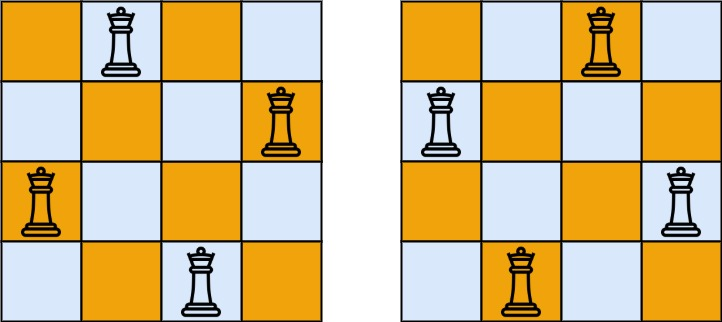### 题目

n 皇后问题 研究的是如何将 n 个皇后放置在 n×n 的棋盘上，并且使皇后彼此之间不能相互攻击。### DFS + 位运算优化

#### DFS 优化

• $vis_C$：$n$ 位二进制，表示可以放置皇后的列编号
• $vis_L$：$2n-1$ 位二进制，表示可以放置皇后的，左下至右上的斜线编号（简称为 $L$ 斜线）
• $vis_R$：$2n-1$ 位二进制，表示可以放置皇后的，左上至右下的斜线编号（简称为 $R$ 斜线）

0 1 2 3
1 2 3 4
2 3 4 5
3 4 5 6

$vis_R$ 的起始位置在整个棋盘左下角

#### 位运算优化

1. 设 $row$ 为当前行编号，$bin(i)$ 为当前列编号 $i$ 表示的二进制数 $1\ll i$，都从 0 开始编号
2. 设置第 $i$ 列不能放置皇后：$vis_C\; \&=\; rev(bin(i))$
3. 设置第 $i$ 列所在的 $L$ 对角线不能放置皇后：$vis_L\; \&=\;rev(bin(i)\ll row)$
4. 设置第 $i$ 列所在的 $R$ 对角线不能放置皇后：$vis_R\; \&=\;rev(bin(i)\ll (n-row-1))$
5. 获取当前状态：$state = vis_C\; \&\; (vis_L\gg row) \;\&\; (vis_R\gg(n-row-1))$